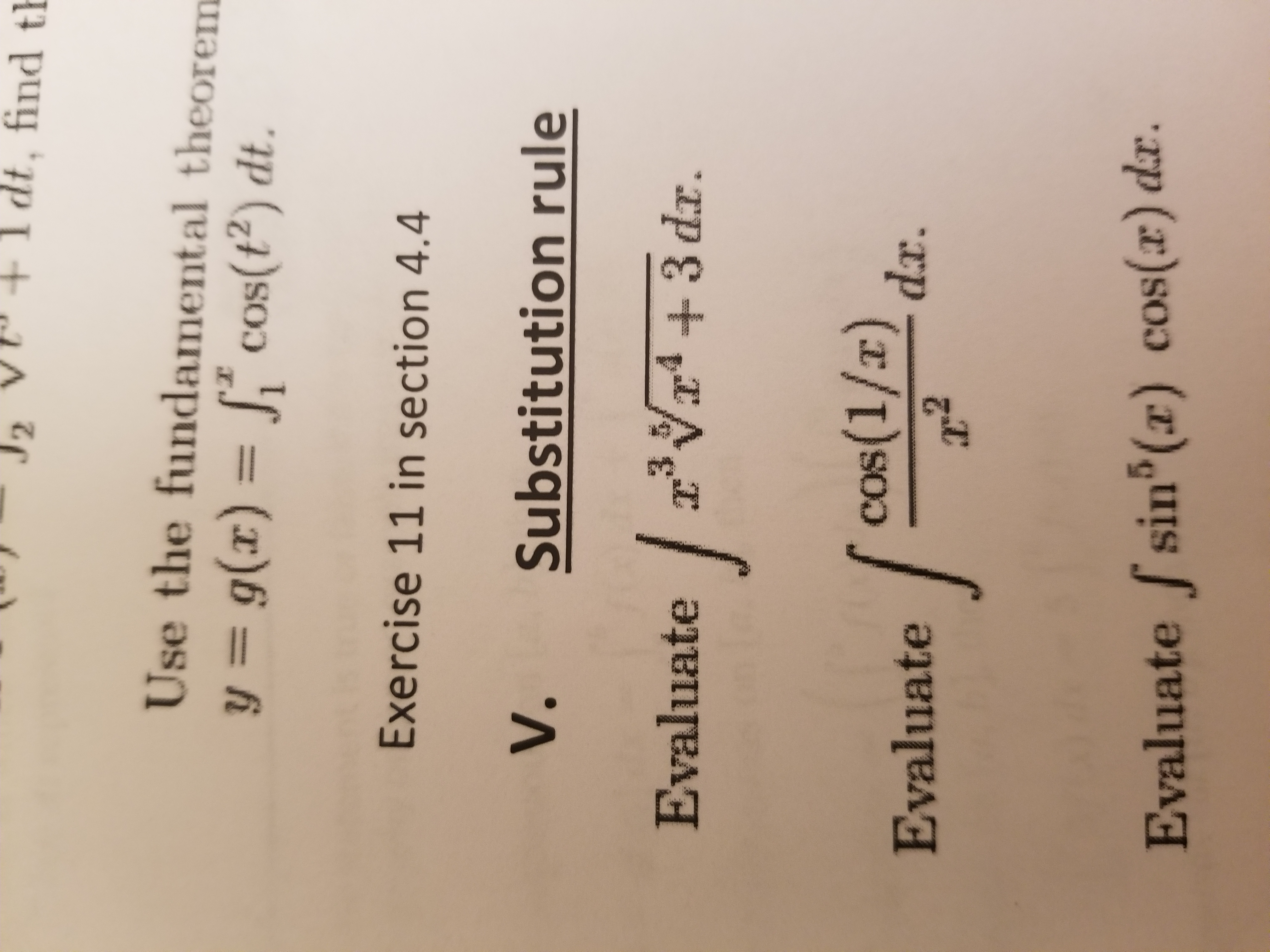# 2dt,find tUse the fundamental theoremg(r) () dtExercise 11 in section 4.4V. Substitution ruleEvaluate34+3d.rcos(1/rEvaluate(/)dxEvaluate sin() cos(z) da.

Question
3 views

I am studying for a quiz and do not understand the substitution rule. can you help work the attached problem for me? If you can only help with one --- please explain the 3rd problem under "V.  Substitution rule".  Thank uou.help_outlineImage Transcriptionclose2 dt, find t Use the fundamental theorem g(r) () dt Exercise 11 in section 4.4 V. Substitution rule Evaluate34+3d.r cos(1/r Evaluate(/) dx Evaluate sin() cos(z) da. fullscreen
check_circle

Step 1

Hey, since there are multiple questions posted, we will answer third question. If you want any other specific question to be answered then please submit that question only or specify the question number in your message.

Step 2

Given:

The integral

Step 3

Calculation:

Compute the given integral as follows.

...

### Want to see the full answer?

See Solution

#### Want to see this answer and more?

Solutions are written by subject experts who are available 24/7. Questions are typically answered within 1 hour.*

See Solution
*Response times may vary by subject and question.
Tagged in

### Other